# Fertilizer

Mr. Gherkin use for fertilization 7% solution of fertilizer. 17 liters of it still left.

How much water must be added to the solution to make only 3% solution?

x =  22.6667 l

### Step-by-step explanation:

7/100•17 = 3/100•(17+x)

3x = 68

x = 68/3 ≈ 22.666667

Our simple equation calculator calculates it.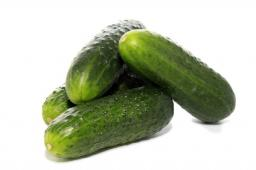Did you find an error or inaccuracy? Feel free to write us. Thank you!Tips to related online calculators
Check out our ratio calculator.
Do you have a linear equation or system of equations and looking for its solution? Or do you have a quadratic equation?
Tip: Our volume units converter will help you with the conversion of volume units.

## Related math problems and questions:

• FertilizerI have 4 liters of 10% fertilizer. How much water do I need to add to have a 2% solution?
• Mixture 2How many liters of water must be added to 7 liters of a 20% solution to obtain a 10% solution?
• Solutions, mixturesHow many liters of 70% solution we must add to 5 liters of 30% solution to give us a 60% solution?
• Solutions, mixturesWe have 2 liters of 20% solution available. How much 70% solution do we need to add to it to get a 30% solution?
• SolutionWhat amount of 80% solution and what amount of water is needed to mix in order to obtain two liters of 60% solution of this mixture?
• Pouring alcohol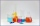100 liters of alcohol has 70% How many liters of water need to be added to have 60% alcohol?
• SolutionsHow much 60% solution and how much 35% solution is needed to create 100 l of 40% solution?
• Vinegar 2How many percentages of vinegar solution will we get if we mix 3.5 liters of 5.8% and 5 liters of 7.6% vinegar?
• Disinfecting solutionHow much distilled water is necessary to pour into 500 ml of 33% hydrogen peroxide solution to obtain 3% disinfecting solution?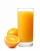Iva added one liter of 100% fruit juice to 3 liters of water. She left two liters of it for Alice and Beata. She added two more liters of water to the remain two liters of lemonade and offered it to other friends. a. What percentage of juice did Alena and
• Water solutionHow many grams of salt should we dissolve in 400 g of water to get a 20% solution?
• Alcohol solutionsWe have to produce 2 liters of 60% alcohol from 55% and 80%. How many of which ones will we use in the solution?
• Copper sulphate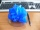How much g of water do we have to add to 240 g of an 84% CuSO4 solution to produce a 60% solution? (Express the mass of crystalline CuSO4 in the original solution and in the resulting solution and compare them. )
• GramsHow many grams of 5% solution do we need to add to 100g of 50% solution to get a 20% solution?
• Hydrochloric acidDetermine the concentration of which must have a solution of hydrochloric acid that mixing 10 l of the solution with 8 liters of 26% solution to get the solution with a concentration of 50%?
• PharmacyAt the pharmacy are in one container 20% solution in the second 50% solution of disinfectant. They need to prepare 4 L of 48-percent solution. What amount of solution from each container is needed to mix?
• Clogging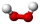How much-distilled water must the pharmacist add to 30g of a 30% hydrogen peroxide solution to obtain a 3% solution to clogging?